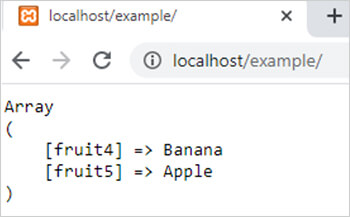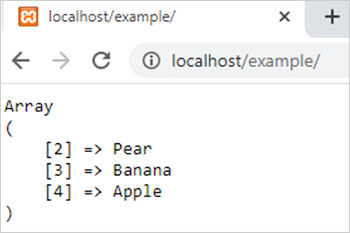array_diff_key() function in php || PHP Functions

array_diff_key() function in php

0 1146

array_diff_key() function of PHP is used to calculate the difference of two or more arrays by comparing the keys of given arrays. It is an inbuilt function of PHP.

Syntax:

array_diff_key (\$arr1,\$arr2,.....,\$arrN);

Here

\$arr1,\$arr2 and \$arrN are arrays.

Example 1:

<?php
\$arr1=array("fruit1"=>"Mango","fruit2"=>"Orange","fruit3"=>"Pear","fruit4"=>"Banana","fruit5"=>"Apple");
\$arr2=array("fruit1"=>"Mango","fruit6"=>"Banana");
\$arr3=array("fruit2"=>"Orange","fruit3"=>"Pear");
\$new_arr=array_diff_key(\$arr1,\$arr2,\$arr3);
echo "<pre>";
print_r(\$new_arr);
?>

Output:Example 2:

<?php
\$arr1=array("Mango","Orange","Pear","Banana","Apple");
\$arr2=array("Mango","Banana");
\$new_arr=array_diff_key(\$arr1,\$arr2);
echo "<pre>";
print_r(\$new_arr);
?>

Output:Share: